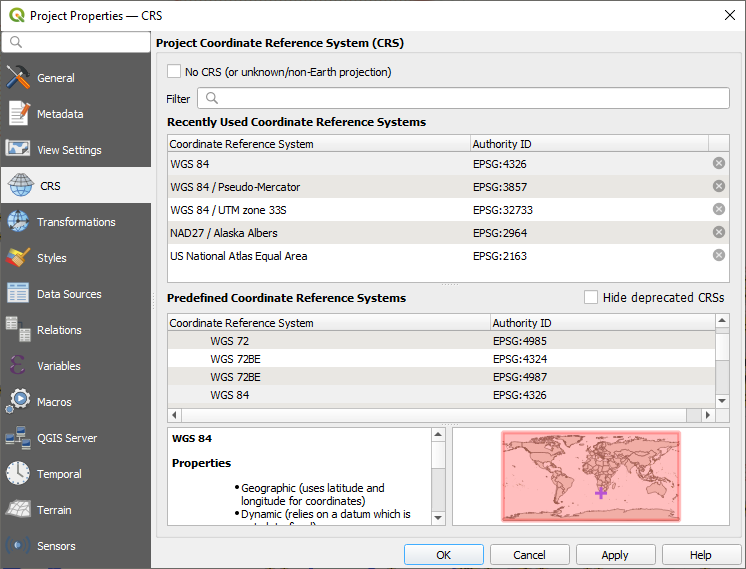# PYQGIS, when we search based on the distance between two points, is the measurement in meters or something else?

I'm new in QGIS and in GIS. I want to calculate distance between two points in meters using WGS 84 coordinates. I'm working with QGIS 2.0.1 Dufour and Python 2.7

The code is the following (based on http://docs.qgis.org/testing/en/docs/pyqgis_developer_cookbook/geometry.html?highlight=measureline#geometry-predicates-and-operations):

``````point1 = QgsPoint(-46.443077,-67.51561)
point2 = QgsPoint(-46.4446,-67.512778)

#Create a measure object
distance = QgsDistanceArea()

#Measure the distance
m = distance.measureLine(point1, point2)
``````

Using these coordinates I'm getting 0.00321554863126 And in Google Maps it is approximately 290m.

Why is it not the same distance, or is the first distance not meters?

• WGS 84 lat/long it isn't in meters. The output is in degrees unit, so you can't compare with Google Maps without a reprojection to a meter-unit Coordinate Reference System. BTW, Google Maps CRS is WGS 84 Pseudo-Mercator – aldo_tapia Dec 26 '17 at 14:45
• Thnx for your help brother , so you want to say that the distance is in degrees , how can i convert it to meters if there is a way in Pyqgis , THNX A LOT – krimou Dec 26 '17 at 20:40
• @krimou For using that class and get acceptable results in meters you need two lines of additional code (with 'setEllipsoid' and 'setEllipsoidalMode' methods). Please see my answer. – xunilk Dec 27 '17 at 11:02

For using that class and get acceptable results in meters you need two lines of additional code (with 'setEllipsoid' and 'setEllipsoidalMode' methods); as it can be observed at follows:

``````point1 = QgsPoint(-46.443077,-67.51561)
point2 = QgsPoint(-46.4446,-67.512778)

#Create a measure object
distance = QgsDistanceArea()
distance.setEllipsoid('WGS84')
distance.setEllipsoidalMode(True) #*

#Measure the distance
m = distance.measureLine(point1, point2)

print m
``````

After running it at Python Console of QGIS result is 322.474631789 meters.

(* From Backwards Incompatible Changes: setEllipsoidalMode() was removed. Ellipsoidal calculations are now enabled whenever a valid ellipsoid() has been set. Set the ellipsoid to 'NONE' to disable ellipsoidal calculations.)

• So when I use the line 'distance.setEllipsoidalMode(True)' means automatically meters? Sorry if is not clear to me – nanunga Jul 13 '18 at 15:46

Yes, you need to set the CRS to one that uses metres before you do any work, unless you want degree units. The length your are receiving is the angle between the two points probably because you have not projected to a CRS that uses metres. You probably are using WGS84 or similar.

https://qgis.org/api/classQgsDistanceArea.html#details

You can check your current units with.

https://qgis.org/api/classQgsDistanceArea.html#abce86a368fff8ffb4507a57d70dcb262

Depending on your latitude you probably will find that converting you vector length to linear units will result in a number close to what your and google are measuring.

You can double check your units in the CRS selection window at the bottom.Notice that this one is US feet: `+units=us-ft`. You would need to find an appropriate one that says `+units=m`.